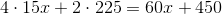# ISEE Upper Level Math : How to find the surface area of a prism

## Example Questions

### Example Question #3 : Solve Problems Involving Area, Volume And Surface Area Of Two And Three Dimensional Objects: Ccss.Math.Content.7.G.B.6

Find the surface area of a non-cubic prism with the following measurements: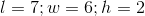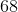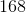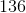Explanation:

The surface area of a non-cubic prism can be determined using the equation: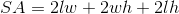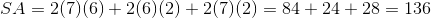### Example Question #1 : Prisms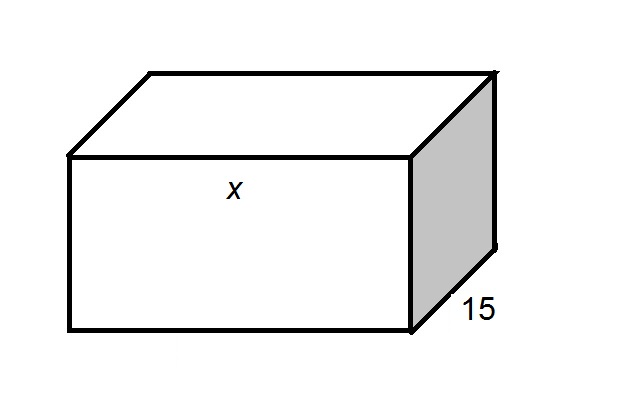The above diagram shows a rectangular solid. The shaded side is a square. In terms of, give the surface area of the box.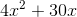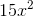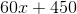Explanation:

A square has four sides of equal length, as seen in the diagram below.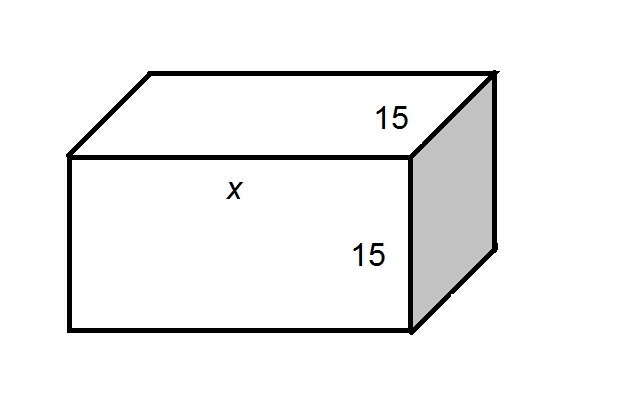All six sides are rectangles, so their areas are equal to the products of their dimensions:

Top, bottom, front, back (four surfaces):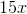Left, right (two surfaces):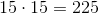The total surface area: